### GE6151 Computer Programming UNIT IV FUNCTIONS AND POINTERS

UNIT IV FUNCTIONS AND POINTERS

Function – definition of function – Declaration of function – Pass by value – Pass by reference –Recursion – Pointers - Definition – Initialization – Pointers arithmetic –

Pointers and arrays- Example Problems.

4. FUNCTION

§ Functions are created when the same process or an algorithm to be repeated several times in various places in the program.

§ Function has a self-contained block of code, that executes certain task. A function has a name, a list of arguments which it takes when called, and the block of code it executes when called.

§ Functions are two types:

ü Built-in / Library function.

ex:) printf(), scanf(), getch(), exit(), etc.

ü User defined function.

User-Defined Functions

Functions defined by the users according to their requirements are called user-defined functions. These functions are used to break down a large program into a small functions.

1. Reduce the source code

2. Easy to maintain and modify

3. It can be called any where in the program.

Body of user defined function:

return_type f_name (argument1,argument 2)

{

local variables; statements; return_type;

The body of user-defined shows that an user-defined functions has following characteristics:

1. Return type

2. Function name

3. Parameter list of arguments

4. Local variables

5. Statements

6. Return value

 S.no C function aspects syntax 1 function definition return_type function_name ( arguments list ) { Body of function; } 2 function call function_name ( arguments list ); 3 function declaration return_type function_name ( argument list );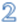Note: The function with return value must be the data type, that is return type and return value must be of same data type.

User defined function has three parts:

1. Declaration part

ret_type f_name (arguments)

2. Calling part f_name(arguments);

3. Definition part

Function Parameters:

Parameters provide the data communication between calling function and called function.

Two types:

§ Actual parameters:

These are the parameters transferred from the calling function[main function] to the called function[user defined function].

§ Formal parameters:

Passing the parameters from the called functions[user defined function] to the calling functions[main function].

Note:

§ Actual parameter – This is the argument which is used in function call.

§ Formal parameter – This is the argument which is used in function definition.

4.1 Categories of User defined function/Function Prototypes:

§ A function prototype declaration consists of the function‟s return type, name and

arguments list.

§ Always terminated with semicolon. The following are the function prototypes:

1. Function without return value and without argument.

2. Function without return value and with argument.

3. Function with return value and with argument.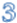4. Function with return value and without argument.

Note: Function with more than one return value will not have return type and return statement in the function definition.

Consider the following example to multiply two numbers:

void main( )

{

int x,y,z;

scanf(“%d%d”, &x,&y);

z=x* y;

printf(“The result is %d”, z);

}

1. Function without return value and without argument

In this prototype, no data transfers takes place between the calling function and the called function. They read data values and print result in the same block.

Syntax:

void f_name(); //function declaration

void f_name (void) //function definition

{

local variables;

statements;

}

void main()

{

f_name(); //function call

}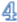The above example can be rewritten by creating function:

void f_mult(); //function definition void f_mult(void )

{

int x,y,z;

scanf(“%d%d”, &x,&y);

z=x* y;

printf(“The result is %d”, z);

}

void main()

{

f_mult();

}

2. Function without return value and with argument

In this prototype, data is transferred from the calling function to called function. The called function receives some data from the calling function and does not send back any values to the calling functions.

Syntax:

void f_name(int x, int y ); //Function declaration

void f_name (int x, int y) //Function Definition //Formal Parameters

{

local variables;

statements;

}

void main()

{

//variable declaration

//input statement

f_name(c, d); //Function call //Actual Parameters

The above example can be rewritten by creating function

void f_mult(int x, int y);

void f_mult(int x, int y )

{

int z;

z=x* y;

printf(“The result is %d”, z);

}

void main()

{

int c, d;

printf(“Enter any two number”); scanf(“%d%d”, &c, &d); f_mult(c, d); //Function call

}

3. Function with return value and without argument

The calling function cannot pass any arguments to the called function but the called function may send some return value to the calling function.

Syntax:

int f_name(); //Function declaration

int f_name (void) //Function Definition

{

local variables; statements; return int;

}

void main()

{

ret_var=f_name(); //Function call

}

The above example can be rewritten by creating function

int f_mult();

int f_mult(void )

{

int x,y,z;

scanf(“%d%d”, &x,&y);

z=x* y;

printf(“The result is %d”, z);

return(z); }

void main()

{

int c; c=f_mult(); getch();

}

4. Function with return value and with argument

In this prototype, the data is transferred between the calling function and called function. The called function receives some data from the calling function and send back a value return to the calling function.

Syntax:

int f_name (int x, int y); //Function declaration

int f_name (int x, int y) //Function definition //Formal Parameters

{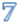local variables; statements; return int;

}

void main()

{

//variable declaration

//Input Statement

ret_value=f_mult(a, b); //Function Call //Actual Parameters

}

The above example can be rewritten by creating function:

int f_mult(int x, int y);

int f_mult(int x, int y)

{

int z;

z=x* y;

printf(“The result is %d”, z);

return(z);

}

void main()

{

int a,b,c;

printf(“Enter any two value:”); scanf(“%d%d”, &a, &b); c=f_mult(a, b);

}

Note:

§ If the return data type of a function is “void”, then, it can‟t return any values to the calling function.

§ If the return data type of the function is other than void such as “int, float, double etc”,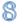then, it can return values to the calling function.

return statement:

It is used to return the information from the function to the calling portion of the program.

Syntax:

return; return(); return(constant); return(variable); return(exp); return(condn_exp);

By default, all the functions return int data type.

Do you know how many values can be return from C functions?

§ Always, only one value can be returned from a function.

§ If you try to return more than one values from a function, only one value will be returned that appears at the right most place of the return statement.

§ For example, if you use “return a,b,c” in your function, value for c only will be returned

and values a, b won‟t be returned to the program.

§ In case, if you want to return more than one values, pointers can be used to directly change the values in address instead of returning those values to the function.

Function Call:

A function can be called by specifying the function_name in the source program with parameters, if presence within the paranthesis.

Syntax: Fn_name(); Fn_name(parameters);

Example:

#include<stdio.h>

#include<conio.h>

int add(int a, int b); //function declaration void main()

{

int x,y,z;

printf(“\n Enter the two values:”);

scanf(“%d%d”,&x,&y);

printf(“The sum is .%d”, z);

}

int add(int a, int b) //Function definition(Formal parameters)

{

int c;

c=a+b;

return(c); //return statement

}